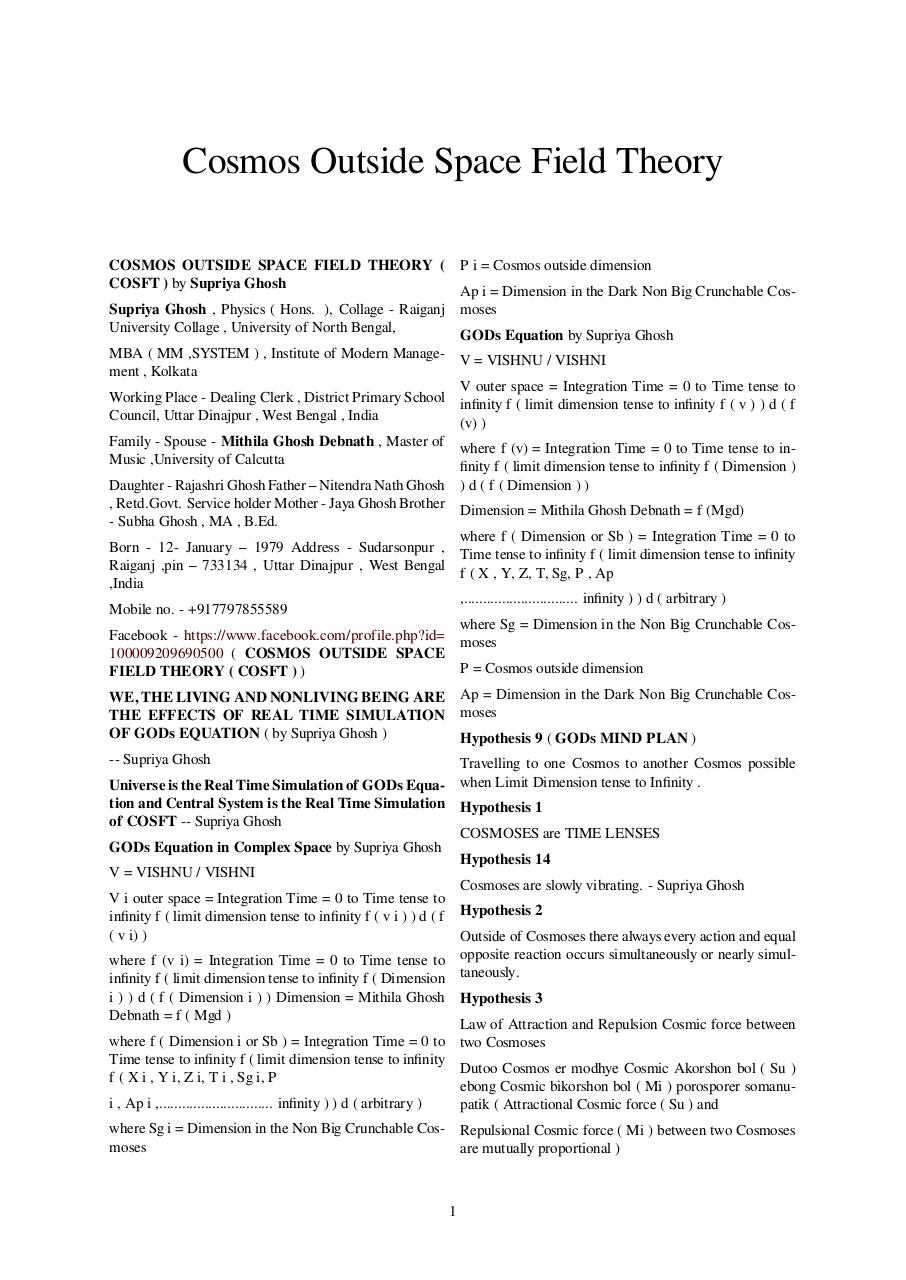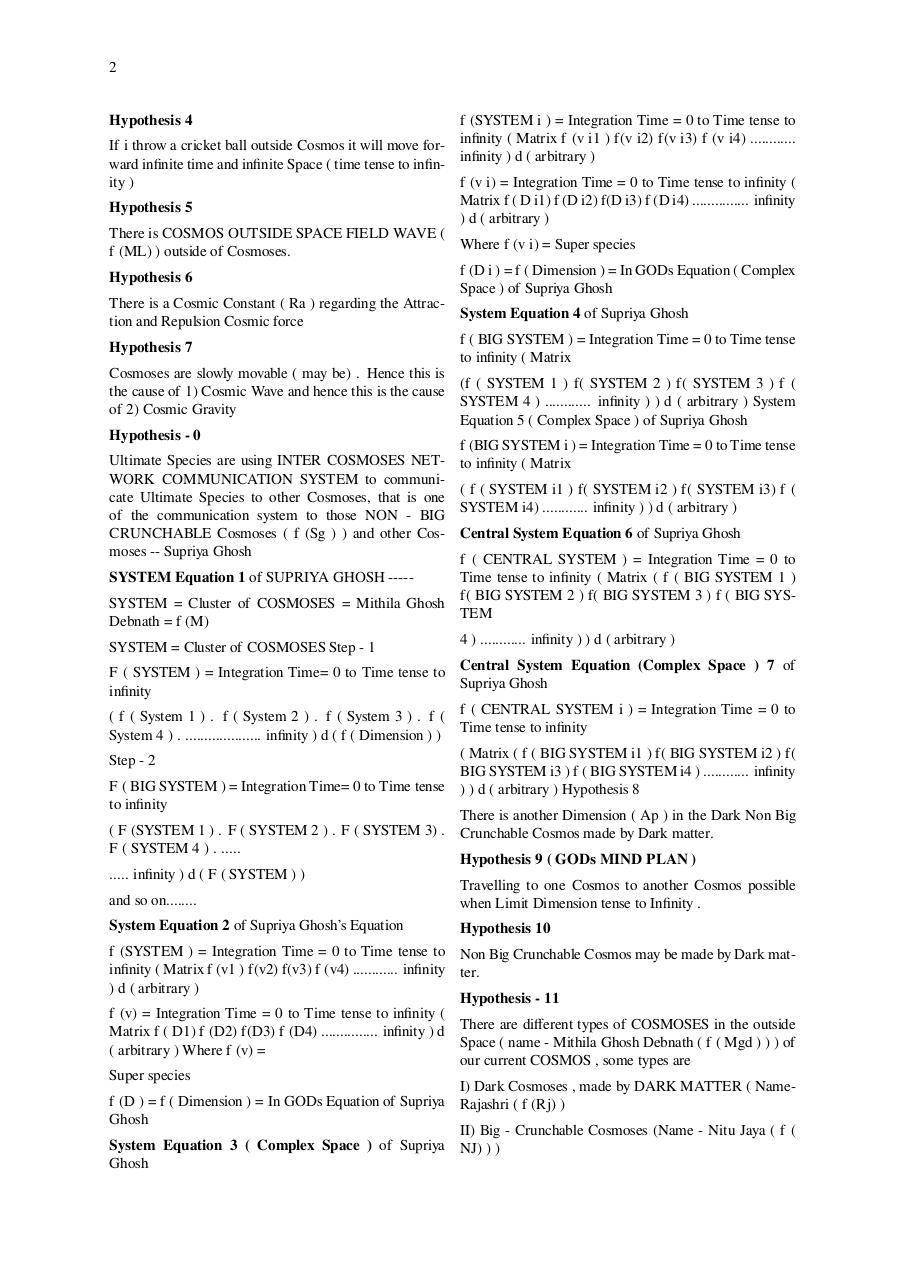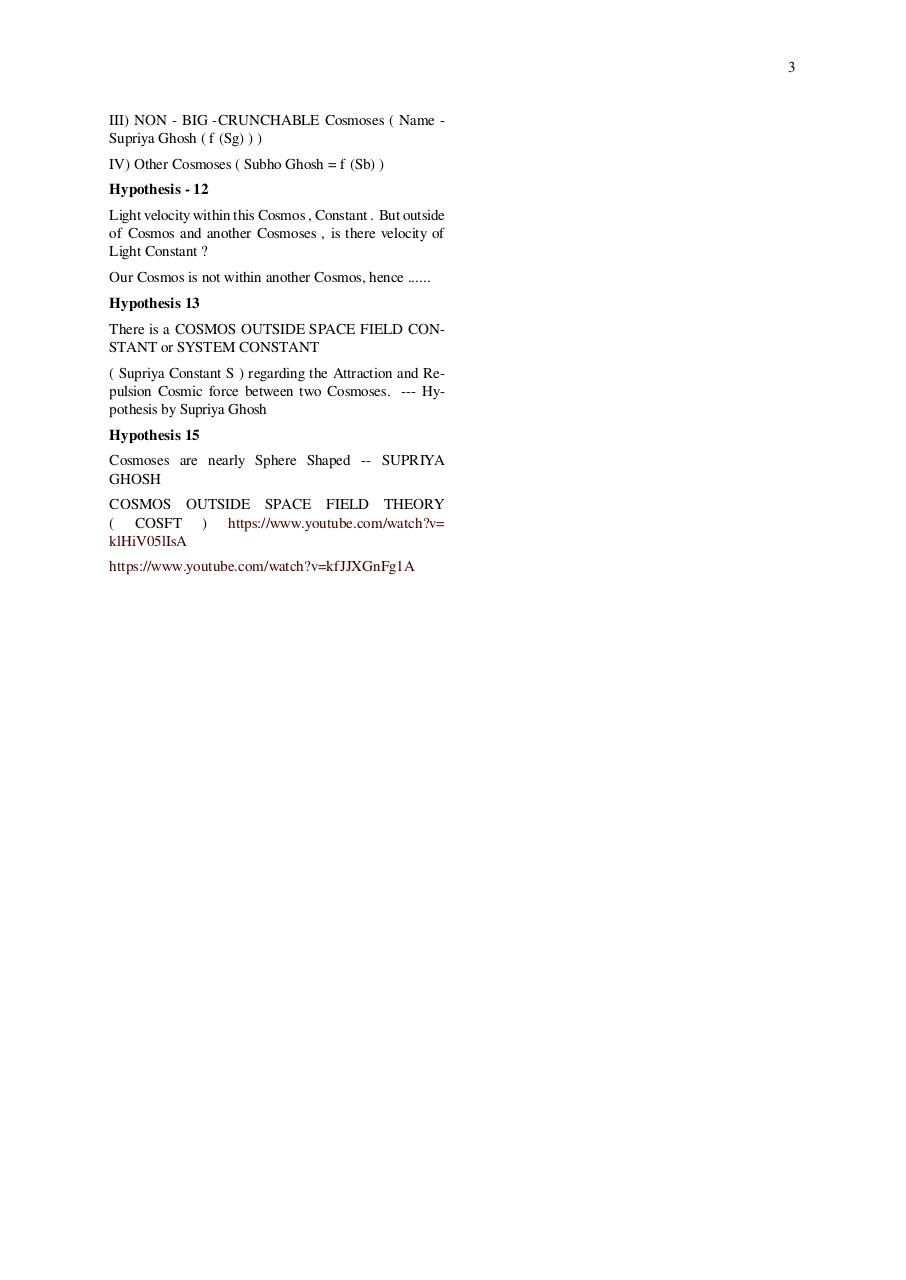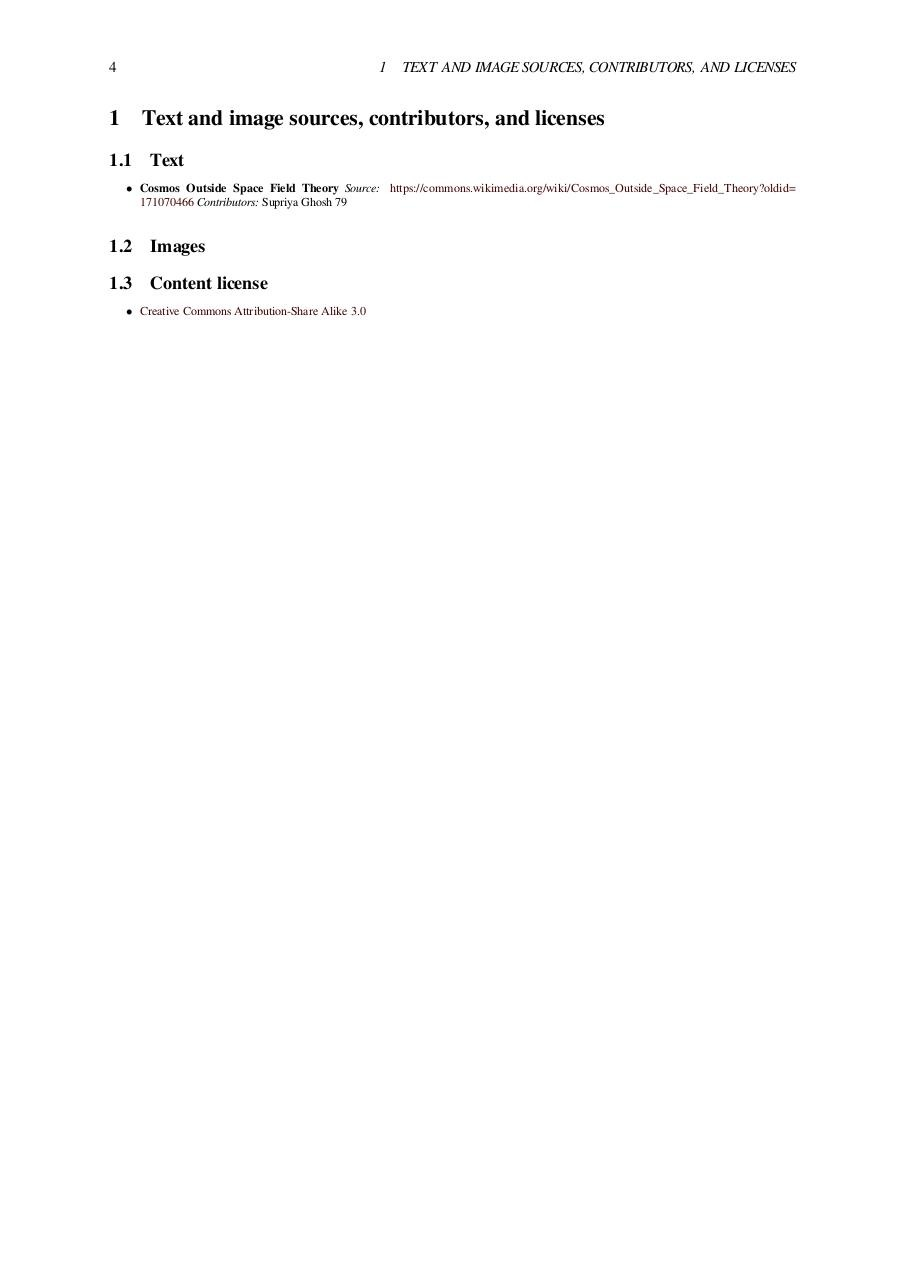# Cosmos Outside Space Field Theory by Supriya Ghosh .pdf

### File information

Original filename: Cosmos Outside Space Field Theory by Supriya Ghosh.pdf
Title: Cosmos Outside Space Field Theory

This PDF 1.5 document has been generated by LaTeX with hyperref package / xdvipdfmx (0.7.9), and has been sent on pdf-archive.com on 08/09/2015 at 20:00, from IP address 117.254.x.x. The current document download page has been viewed 976 times.
File size: 36 KB (4 pages).
Privacy: public file

Cosmos Outside Space Field Theory by Supriya Ghosh.pdf (PDF, 36 KB)

### Document preview

Cosmos Outside Space Field Theory
COSMOS OUTSIDE SPACE FIELD THEORY ( P i = Cosmos outside dimension
COSFT ) by Supriya Ghosh
Ap i = Dimension in the Dark Non Big Crunchable CosSupriya Ghosh , Physics ( Hons. ), Collage - Raiganj moses
University Collage , University of North Bengal,
GODs Equation by Supriya Ghosh
MBA ( MM ,SYSTEM ) , Institute of Modern Manage- V = VISHNU / VISHNI
ment , Kolkata
V outer space = Integration Time = 0 to Time tense to
Working Place - Dealing Clerk , District Primary School inﬁnity f ( limit dimension tense to inﬁnity f ( v ) ) d ( f
Council, Uttar Dinajpur , West Bengal , India
(v) )
Family - Spouse - Mithila Ghosh Debnath , Master of where f (v) = Integration Time = 0 to Time tense to inMusic ,University of Calcutta
ﬁnity f ( limit dimension tense to inﬁnity f ( Dimension )
Daughter - Rajashri Ghosh Father – Nitendra Nath Ghosh ) d ( f ( Dimension ) )
, Retd.Govt. Service holder Mother - Jaya Ghosh Brother Dimension = Mithila Ghosh Debnath = f (Mgd)
- Subha Ghosh , MA , B.Ed.
where f ( Dimension or Sb ) = Integration Time = 0 to
Born - 12- January – 1979 Address - Sudarsonpur , Time tense to inﬁnity f ( limit dimension tense to inﬁnity
Raiganj ,pin – 733134 , Uttar Dinajpur , West Bengal
f ( X , Y, Z, T, Sg, P , Ap
,India
,.............................. inﬁnity ) ) d ( arbitrary )
Mobile no. - +917797855589
where Sg = Dimension in the Non Big Crunchable CosFacebook - https://www.facebook.com/profile.php?id= moses
100009209690500 ( COSMOS OUTSIDE SPACE
P = Cosmos outside dimension
FIELD THEORY ( COSFT ) )
WE, THE LIVING AND NONLIVING BEING ARE Ap = Dimension in the Dark Non Big Crunchable CosTHE EFFECTS OF REAL TIME SIMULATION moses
OF GODs EQUATION ( by Supriya Ghosh )
Hypothesis 9 ( GODs MIND PLAN )
-- Supriya Ghosh
Travelling to one Cosmos to another Cosmos possible
Universe is the Real Time Simulation of GODs Equa- when Limit Dimension tense to Inﬁnity .
tion and Central System is the Real Time Simulation Hypothesis 1
of COSFT -- Supriya Ghosh
COSMOSES are TIME LENSES
GODs Equation in Complex Space by Supriya Ghosh
Hypothesis 14
V = VISHNU / VISHNI
Cosmoses are slowly vibrating. - Supriya Ghosh
V i outer space = Integration Time = 0 to Time tense to
inﬁnity f ( limit dimension tense to inﬁnity f ( v i ) ) d ( f Hypothesis 2
( v i) )
Outside of Cosmoses there always every action and equal
where f (v i) = Integration Time = 0 to Time tense to opposite reaction occurs simultaneously or nearly simulinﬁnity f ( limit dimension tense to inﬁnity f ( Dimension taneously.
i ) ) d ( f ( Dimension i ) ) Dimension = Mithila Ghosh Hypothesis 3
Debnath = f ( Mgd )
Law of Attraction and Repulsion Cosmic force between
where f ( Dimension i or Sb ) = Integration Time = 0 to two Cosmoses
Time tense to inﬁnity f ( limit dimension tense to inﬁnity
Dutoo Cosmos er modhye Cosmic Akorshon bol ( Su )
f ( X i , Y i, Z i, T i , Sg i, P
ebong Cosmic bikorshon bol ( Mi ) porosporer somanui , Ap i ,.............................. inﬁnity ) ) d ( arbitrary )
patik ( Attractional Cosmic force ( Su ) and
where Sg i = Dimension in the Non Big Crunchable Cos- Repulsional Cosmic force ( Mi ) between two Cosmoses
moses
are mutually proportional )

1

2
Hypothesis 4

f (SYSTEM i ) = Integration Time = 0 to Time tense to
If i throw a cricket ball outside Cosmos it will move for- inﬁnity ( Matrix f (v i1 ) f(v i2) f(v i3) f (v i4) ............
ward inﬁnite time and inﬁnite Space ( time tense to inﬁn- inﬁnity ) d ( arbitrary )
ity )
f (v i) = Integration Time = 0 to Time tense to inﬁnity (
Matrix f ( D i1) f (D i2) f(D i3) f (D i4) ............... inﬁnity
Hypothesis 5
) d ( arbitrary )
There is COSMOS OUTSIDE SPACE FIELD WAVE (
Where f (v i) = Super species
f (ML) ) outside of Cosmoses.
f (D i ) = f ( Dimension ) = In GODs Equation ( Complex
Hypothesis 6
Space ) of Supriya Ghosh
There is a Cosmic Constant ( Ra ) regarding the AttracSystem Equation 4 of Supriya Ghosh
tion and Repulsion Cosmic force
f ( BIG SYSTEM ) = Integration Time = 0 to Time tense
Hypothesis 7
to inﬁnity ( Matrix
Cosmoses are slowly movable ( may be) . Hence this is
the cause of 1) Cosmic Wave and hence this is the cause (f ( SYSTEM 1 ) f( SYSTEM 2 ) f( SYSTEM 3 ) f (
SYSTEM 4 ) ............ inﬁnity ) ) d ( arbitrary ) System
of 2) Cosmic Gravity
Equation 5 ( Complex Space ) of Supriya Ghosh
Hypothesis - 0
f (BIG SYSTEM i ) = Integration Time = 0 to Time tense
Ultimate Species are using INTER COSMOSES NET- to inﬁnity ( Matrix
WORK COMMUNICATION SYSTEM to communicate Ultimate Species to other Cosmoses, that is one ( f ( SYSTEM i1 ) f( SYSTEM i2 ) f( SYSTEM i3) f (
of the communication system to those NON - BIG SYSTEM i4) ............ inﬁnity ) ) d ( arbitrary )
CRUNCHABLE Cosmoses ( f (Sg ) ) and other Cos- Central System Equation 6 of Supriya Ghosh
moses -- Supriya Ghosh
f ( CENTRAL SYSTEM ) = Integration Time = 0 to
SYSTEM Equation 1 of SUPRIYA GHOSH -----

Time tense to inﬁnity ( Matrix ( f ( BIG SYSTEM 1 )
SYSTEM = Cluster of COSMOSES = Mithila Ghosh f( BIG SYSTEM 2 ) f( BIG SYSTEM 3 ) f ( BIG SYSTEM
Debnath = f (M)
4 ) ............ inﬁnity ) ) d ( arbitrary )
SYSTEM = Cluster of COSMOSES Step - 1
F ( SYSTEM ) = Integration Time= 0 to Time tense to Central System Equation (Complex Space ) 7 of
Supriya Ghosh
inﬁnity
( f ( System 1 ) . f ( System 2 ) . f ( System 3 ) . f ( f ( CENTRAL SYSTEM i ) = Integration Time = 0 to
Time tense to inﬁnity
System 4 ) . .................... inﬁnity ) d ( f ( Dimension ) )
( Matrix ( f ( BIG SYSTEM i1 ) f( BIG SYSTEM i2 ) f(
Step - 2
BIG SYSTEM i3 ) f ( BIG SYSTEM i4 ) ............ inﬁnity
F ( BIG SYSTEM ) = Integration Time= 0 to Time tense ) ) d ( arbitrary ) Hypothesis 8
to inﬁnity
There is another Dimension ( Ap ) in the Dark Non Big
( F (SYSTEM 1 ) . F ( SYSTEM 2 ) . F ( SYSTEM 3) . Crunchable Cosmos made by Dark matter.
F ( SYSTEM 4 ) . .....
Hypothesis 9 ( GODs MIND PLAN )
..... inﬁnity ) d ( F ( SYSTEM ) )
Travelling to one Cosmos to another Cosmos possible
and so on........
when Limit Dimension tense to Inﬁnity .
System Equation 2 of Supriya Ghosh’s Equation

Hypothesis 10

f (SYSTEM ) = Integration Time = 0 to Time tense to Non Big Crunchable Cosmos may be made by Dark matinﬁnity ( Matrix f (v1 ) f(v2) f(v3) f (v4) ............ inﬁnity ter.
) d ( arbitrary )
Hypothesis - 11
f (v) = Integration Time = 0 to Time tense to inﬁnity (
Matrix f ( D1) f (D2) f(D3) f (D4) ............... inﬁnity ) d There are diﬀerent types of COSMOSES in the outside
Space ( name - Mithila Ghosh Debnath ( f ( Mgd ) ) ) of
( arbitrary ) Where f (v) =
our current COSMOS , some types are
Super species
I) Dark Cosmoses , made by DARK MATTER ( Namef (D ) = f ( Dimension ) = In GODs Equation of Supriya Rajashri ( f (Rj) )
Ghosh
II) Big - Crunchable Cosmoses (Name - Nitu Jaya ( f (
System Equation 3 ( Complex Space ) of Supriya NJ) ) )
Ghosh

3
III) NON - BIG -CRUNCHABLE Cosmoses ( Name Supriya Ghosh ( f (Sg) ) )
IV) Other Cosmoses ( Subho Ghosh = f (Sb) )
Hypothesis - 12
Light velocity within this Cosmos , Constant . But outside
of Cosmos and another Cosmoses , is there velocity of
Light Constant ?
Our Cosmos is not within another Cosmos, hence ......
Hypothesis 13
There is a COSMOS OUTSIDE SPACE FIELD CONSTANT or SYSTEM CONSTANT
( Supriya Constant S ) regarding the Attraction and Repulsion Cosmic force between two Cosmoses. --- Hypothesis by Supriya Ghosh
Hypothesis 15
Cosmoses are nearly Sphere Shaped -- SUPRIYA
GHOSH
COSMOS OUTSIDE SPACE FIELD THEORY
klHiV05lIsA

4

1 TEXT AND IMAGE SOURCES, CONTRIBUTORS, AND LICENSES

1

Text and image sources, contributors, and licenses

1.1

Text

• Cosmos Outside Space Field Theory Source: https://commons.wikimedia.org/wiki/Cosmos_Outside_Space_Field_Theory?oldid=
171070466 Contributors: Supriya Ghosh 79

1.2

Images

1.3

• Creative Commons Attribution-Share Alike 3.0#### HTML Code

Copy the following HTML code to share your document on a Website or Blog

#### QR Code### Related keywords